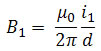Electricity & Megnetism

# Magnetic force between parallel wires

Parallel wires carrying electric current can experience magnetic attraction or repulsion. This will depend on the directions of force and current.

Ampère did studies related to the magnetic force produced between two wires that conduct electrical energy. In his studies, he was able to determine the strength of the magnetic field produced by this electric current . As we can see in the figure below, the magnetic force between two parallel wires and separated by a distance d can be determined as follows

Initially, we must calculate the strength of the magnetic field B 1 at the position of wire 2. Thus, the field produced by the current i 1 is:Then we can calculate the magnitude of the magnetic force acting on wire 2 using the following equation: F 1 = B 1 .i 2 .L. In this equation, L is the length of the wire. Thus, we can see that the magnetic force acting on wire 2 is given by the following relationship:

In vacuum, we have that µ = 4π.10-7 Tm/A.

Thus, we can say that the same effect occurs for the magnetic field generated by wire 2. Thus, the magnetic field created by current i 2 , at the position of wire 1, also produces a force on current i 1 . This force has the same magnitude as the force F 2 , but has the opposite direction. These two forces form an action-reaction pair.
Using the right-hand rule, we can see that if the currents are in the same direction, the magnetic force between the wires will be attractive. If the currents have opposite directions, the force will be repulsive between the wires.